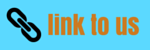# System of particles and Collision

## (14) Head on inelastic collision of two particles which stick together

A) In laboraratory frame of refrence

• Consider two particles whose masses are m1 and m2 .Let u1 and u2 be the respective velocities before collision
• Let both the particles stick together after collision and moves with the same velocity v .Then from law of conservation of linear momentum
m1u1 +m2u2=(m1+m2)v
or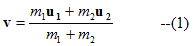• If we consider second particle to be stationary or at rest then u2=0
then
m1u1 =(m1+m2)
or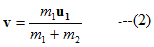Hence |v| < |u1|

• Kinetic energy before collision is
KE1=(1/2)m1u12

After collison KE of the system is
KE2=(1/2)(m1+m2)v2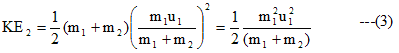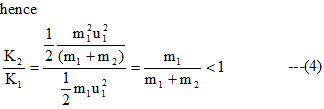Hence from equation we come to know that K2 < K1 hence energy loss would be there after thye collision of the particles

B) In center of mass frame of refrence:-

• Velocity of center of mass is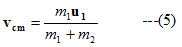when second particle is at rest.
It is clear that Center of masss frame of refrence moves with velocity vcm w.r.t laboratory frame of refrence.
Hence velocity of particle having mass m1 in C-frame of refrence is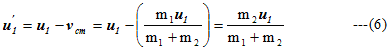And velocity of particle having mass m2 in C-frame of refrence would be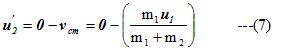• let v' be the joint velocity of the particles which stick to each other after the collision in center of mass frame of refrence .So by the law of conservation of linear momentum

(m1+m2)v'=m1u1' +m2u2'

Substituting the values of u1' and u2' we find
(m1+m2)v'=0
but (m1+m2) can be zero so
v'=0

Therefore after collision total momentum of the particles comes out to be zero.Because both the particles stick to each other after collision so the velocity of joint particle mass i.e(m1+m2) would be zero.Therefore in center of mass frame of refrence ,particle which stick together will remain stationary.

## (15) Deflection of an moving particle by a particle at rest during perfectly elastic collision or elastic collision in two dimension

A) In labaoratory frame of refrence

• Let m1 and m2 be the two mass particle in a laboratory frame of refrence and m1 collide with m2 which is initailly is at rest.Let the velocity of mass m1 before collison be u1 and after the collison it moves with a velocity v1 and is delfected by the angle θ1 withs its incident direction and
m2 after the collision moves with the velocity v2 and it is deflected by an angle θ2 with its incident direction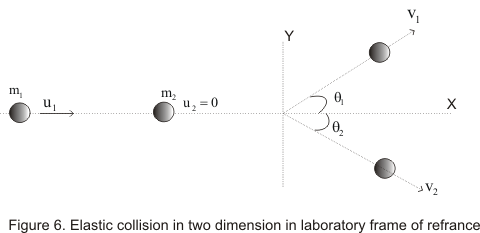• From law of conservation of linear momentum ,for components along x-axis
m1u1=m1v1cosθ1 +m2v2cosθ2 ---(1)
For components along y-axis
0=m1v1sinθ1 -m2v2sinθ2 --(2)
And from law of conservation of energy
(1/2)m1u12=(1/2)m1v12+(1/2)m2v22 ---(3)

• Analysing above equations we come to know that we have to find values of four unknown quantities v1,v212 with the help of above three equations which is impossible as we need to have atleast four equations for finding out the values of four unknown quantities .Hence this problem can be solved in C frame of refrence

B) In center of mass frame of refrence

• Velocity of the center of mass wrt to L -frame of refrence is equation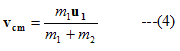• In C frame of refrence ,the center of mass remain stationary ,the velocity of mass m1 before collision w.r.t C-frame of refrence is
u1'=u1 - vcm                   (5)
And that of mass m2 is
u2'=0 - vcm = - vcm                   (6)

After the collision velocites of m1 and m2 in C-frame of refrence would be
v1'=v1 - vcm                   (7)
v2'=v2 - vcm                   (8)

Since center of mass remains stationary in C-frame of refrence hence total momentum would be zero.Therefore the momentum of both the massed would be equal and in opposite direction
So
m1u1' = -m2u2'                     --(9)
m1v1' = -m2v2'                    --(10)

The above equation can be proved like this
m1u1' = m1(u1 - vcm)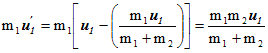and -m2u2' = m2vcm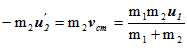From the above equations
m1u1' = -m2u2'
Similarly we can prove that

m1v1' = -m2v2'

• It is clear from the above equations that after collisions the velocity of the particles i.e v1',v2' would be in opposite direction to each other and make same angles with the direction of the intial velocities of the particles as shown fig: (b)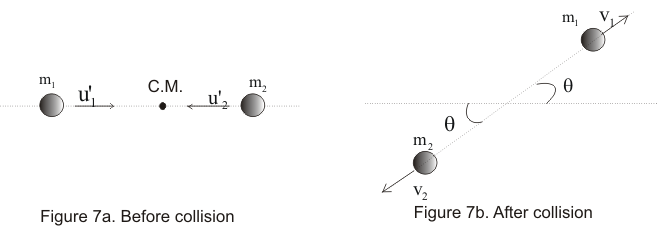• From equation (9) and (10)
u2'=(m1/m2)u1'

and v2'=(m1/m2)b>v1'

• From the law of conservation of energy in this frame of refrence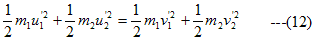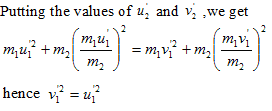• From which |v1'|=|u1'| and |v2'|=|u2'|.
• From these equations we came to know that magnitude of the velocities in C-frame of refrance , when the collision is elastic, does not change but their direction could change after collision.